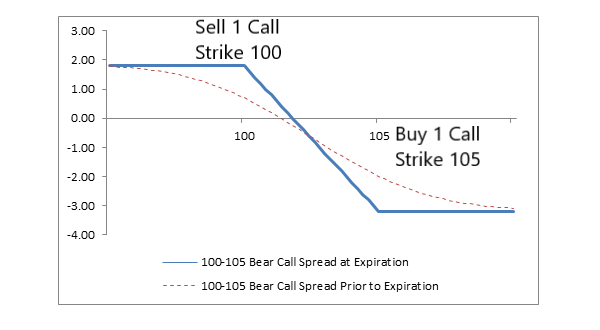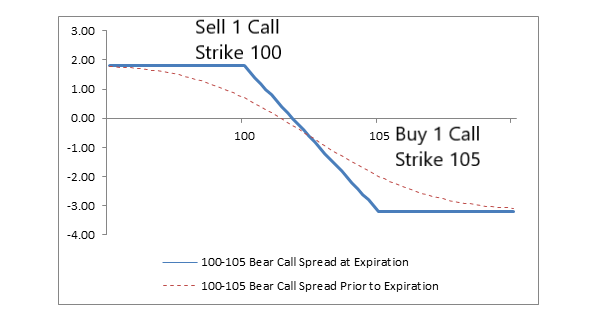### Goal

To profit from neutral to bearish price action in the underlying stock.

A bear call spread consists of one short call with a lower strike price and one long call with a higher strike price.

Both calls have the same underlying stock and the same expiration date.

A bear call spread is established for a net credit (or net amount received) and profits from either a declining stock price or from time erosion or from both.

Potential profit is limited to the net premium received less commissions and potential loss is limited if the stock price rises above the strike price of the long call.

### Maximum profit

Potential profit is limited to the net premium received less commissions, and this profit is realized if the stock price is at or below the strike price of the short call (lower strike) at expiration and both calls expire worthless.

### Maximum risk

The maximum risk is equal to the difference between the strike prices minus the net credit received including commissions.

In the example above, the difference between the strike prices is 5.00 (105.00 – 100.00 = 5.00), and the net credit is 1.80 (3.30 – 1.50 = 1.80).

The maximum risk, therefore, is 3.20 (5.00 – 1.80 = 3.20) per share less commissions.

This maximum risk is realized if the stock price is at or above the strike price of the long call at expiration.

Short calls are generally assigned at expiration when the stock price is above the strike price. However, there is a possibility of early assignment. See below.

### Appropriate market forecast

A bear call spread earns the maximum profit when the price of the underlying stock is below the strike price of the short call (lower strike price) at expiration.

Therefore, the ideal forecast is “neutral to bearish price action.”

### Strategy

The bear call spreads is a strategy that “collects option premium and limits risk at the same time.” They profit from both time decay and falling stock prices.

A bear call spread is the strategy of choice when the forecast is for neutral to falling prices and there is a desire to limit risk.

### Impact of stock price change

A bear call spread benefits when the underlying price falls and is hurt when it rises.

This means that the position has a “net negative delta.”

Delta estimates how much an option price will change as the stock price changes, and the change in option price is generally less than dollar-for-dollar with the change in stock price.

Also, because a bear call spread consists of one short call and one long call, the net delta changes very little as the stock price changes and time to expiration is unchanged.

In the language of options, this is a “near-zero gamma.”

Gamma estimates how much the delta of a position changes as the stock price changes.

### Impact of change in volatility

Volatility is a measure of how much a stock price fluctuates in percentage terms, and volatility is a factor in option prices.

As volatility rises, option prices tend to rise if other factors such as stock price and time to expiration remain constant.

Since a bear call spread consists of one short call and one long call, the price of a bear call spread changes very little when volatility changes and other factors remain constant.

In the language of options, this is a “near-zero vega.” Vega estimates how much an option price changes as the level of volatility changes and other factors are unchanged.

### Impact of time

The time value portion of an option’s total price decreases as expiration approaches. This is known as time erosion.

Since a bear call spread consists of one short call and one long call, the sensitivity to time erosion depends on the relationship of the stock price to the strike prices of the spread.

If the stock price is “close to” or below the strike price of the short call (lower strike price), then the price of the bear call spread decreases (and makes money) with passing of time.

This happens because the short call is closest to the money and erodes faster than the long call.

However, if the stock price is “close to” or above the strike price of the long call (higher strike price), then the price of the bear call spread increases (and loses money) with passing time.

This happens because the long call is now closer to the money and erodes faster than the short call.

If the stock price is half-way between the strike prices, then time erosion has little effect on the price of a bear call spread, because both the short call and the long call erode at approximately the same rate.